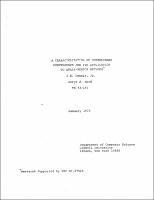## A Characterization of Superlinear Convergence and its Application to Quasi-Newton Methods##### Abstract
Let F be a mapping from real n-dimensional Euclidean space into itself. Most practical algorithms for finding a zero of F are of the form $x_{k+1} = x_{k} - B_{k}^{-1_{Fx_{k}}}$ where $\{B_{k}\}$ is a sequence of non-singular matrices. The main result of this paper is a characterization theorem for the superlinear convergence to a zero of F of sequences of the above form. This result is then used to give a unified treatment of the results on the superlinear convergence of the Davidon-Fletcher-Powell method obtained by Powell for the case in which exact line searches are used, and by Broyden, Dennis, and More for the case without line searches. As a by-product, several results on the asymptotic behavior of the sequence $\{B_{k}\}$ are obtained. An interesting aspect of these results is that superlinear convergence is obtained without any consistency conditions; i.e. without requiring that the sequence $\{B_{k}\}$ converge to the Jacobian.
1973-01
##### Publisher
Cornell University
##### Keywords
computer science; technical report
##### Previously Published As
http://techreports.library.cornell.edu:8081/Dienst/UI/1.0/Display/cul.cs/TR73-157
technical report